## ML Aggarwal Class 10 Solutions for ICSE Maths Chapter 7 Ratio and Proportion Ex 7.2

These Solutions are part of ML Aggarwal Class 10 Solutions for ICSE Maths. Here we have given ML Aggarwal Class 10 Solutions for ICSE Maths Chapter 7 Ratio and Proportion Ex 7.2

More Exercises

Question 1.
Find the value of x in the following proportions :
(i) 10 : 35 = x : 42
(ii) 3 : x = 24 : 2
(iii) 2.5 : 1.5 = x : 3
(iv) x : 50 :: 3 : 2
Solution:
(i) 10 : 35 = x : 42
⇒ 35 × x = 10 × 42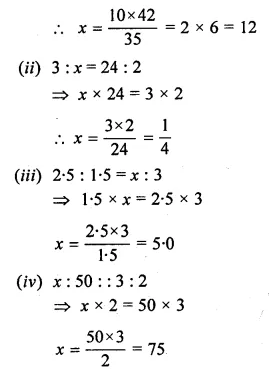Question 2.
Find the fourth proportional to
(i) 3, 12, 15
(ii) $$\frac { 1 }{ 3 } ,\frac { 1 }{ 4 } ,\frac { 1 }{ 5 }$$
(iii) 1.5, 2.5, 4.5
(iv) 9.6 kg, 7.2 kg, 28.8 kg
Solution:
(i) Let fourth proportional to
3, 12, 15 be x.
then 3 : 12 :: 15 : x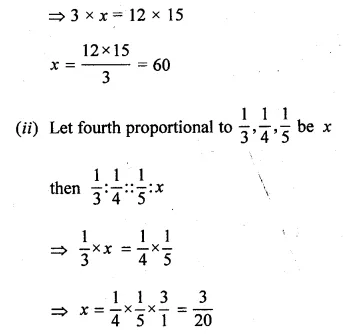Question 3.
Find the third proportional to
(i) 5, 10
(ii) 0.24, 0.6
(iii) Rs. 3, Rs. 12
(iv) $$5 \frac { 1 }{ 4 }$$ and 7.
Solution:
(i) Let x be the third proportional to 5, 10,
then 5 : 10 :: 10 : x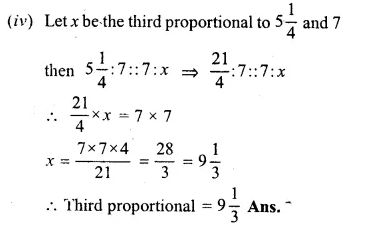Question 4.
Find the mean proportion of:
(i) 5 and 80
(ii) $$\\ \frac { 1 }{ 12 }$$ and $$\\ \frac { 1 }{ 75 }$$
(iii) 8.1 and 2.5
(iv) (a – b) and (a³ – a²b), a> b
Solution:
(i) Let x be the mean proportion of 5 and 80 ,
then 5 : x : : x : 80
x² = 5 x 80
⇒ x = $$\sqrt { 5\times 80 } =\sqrt { 400 }$$ = 20
x = 20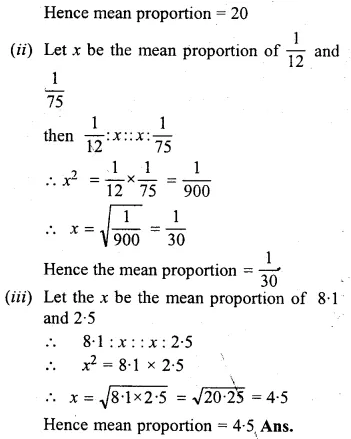Question 5.
If a, 12, 16 and b are in continued proportion find a and b.
Solution:
∵ a, 12, 16, b are in continued proportion, thenQuestion 6.
What number must be added to each of the numbers 5, 11, 19 and 37 so that they are in proportion ? (2009)
Solution:
Let x be added to 5, 11, 19 and 37 to make them in proportion.
5 + x : 11 + x : : 19 + x : 37 + x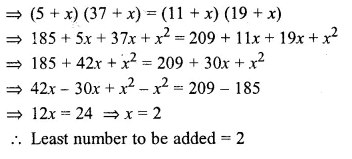Question 7.
What number should be subtracted from each of the numbers 23, 30, 57 and 78 so that the remainders are in proportion ? (2004)
Solution:
Let x be subtracted from each term, then
23 – x, 30 – x, 57 – x and 78 – x are proportional
23 – x : 30 – x : : 57 – x : 78 – x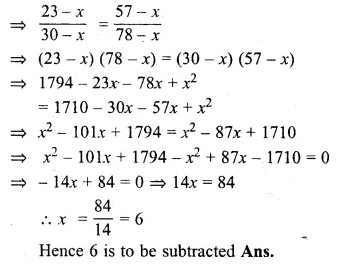Question 8.
If 2x – 1, 5x – 6, 6x + 2 and 15x – 9 are in proportion, find the value of x.
Solution:
∵ 2x – 1, 5x – 6, 6x + 2 and 15x – 9 are in proportion.
then (2x – 1) (15x – 9) = (5x – 6) (6x + 2)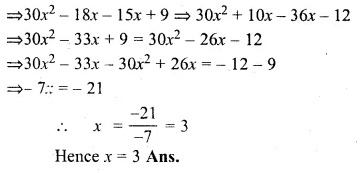Question 9.
If x + 5 is the mean proportion between x + 2 and x + 9, find the value of x.
Solution:
∵ x + 5 is the mean proportion between x + 2 and x + 9, then
(x + 5)² = (x + 2) (x + 9)
⇒ x² + 10x + 25 = x² + 11x + 18
⇒ x² + 10x – x² – 11x = 18 – 25
⇒ – x = – 7
∵ x = 7 Ans.

Question 10.
What number must be added to each of the numbers 16, 26 and 40 so that the resulting numbers may be in continued proportion?
Solution:
Let x be added to each number then
16 + x, 26 + x and 40 + x
are in continued proportion.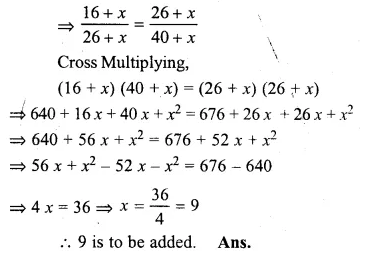Question 11.
Find two numbers such that the mean proportional between them is 28 and the third proportional to them is 224.
Solution:
Let the two numbers are a and b.
∵ 28 is the mean proportional
∵ a : 28 : : 28 : b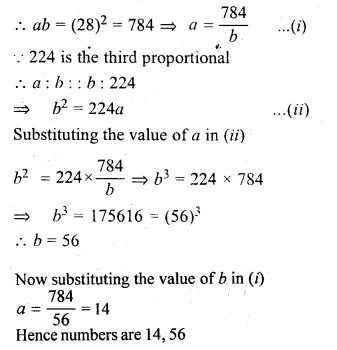Question 12.
If b is the mean proportional between a and c, prove that a, c, a² + b², and b² + c² are proportional.
Solution:
∵ b is the mean proportional between a and c, then,
b² = a × c ⇒ b² = ac …(i)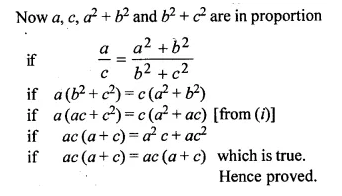Question 13.
If b is the mean proportional between a and c, prove that (ab + bc) is the mean proportional between (a² + b²) and (b² + c²).
Solution:
b is the mean proportional between a and c then
b² = ac …(i)
Now if (ab + bc) is the mean proportional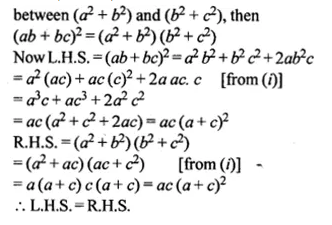Question 14.
If y is mean proportional between x and z, prove that
xyz (x + y + z)³ = (xy + yz + zx)³.
Solution:
∵ y is the mean proportional between
x and z, then
y² = xz …(i)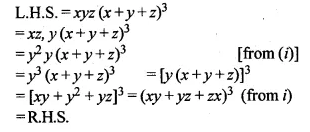Question 15.
If a + c = mb and $$\frac { 1 }{ b } +\frac { 1 }{ d } =\frac { m }{ c }$$, prove that a, b, c and d are in proportion.
Solution:
a + c = mb and $$\frac { 1 }{ b } +\frac { 1 }{ d } =\frac { m }{ c }$$
a + c = mb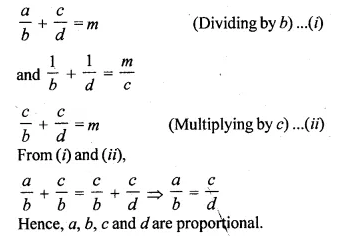Question 16.
If $$\frac { x }{ a } =\frac { y }{ b } =\frac { z }{ c }$$, prove that
(i)$$\frac { { x }^{ 3 } }{ { a }^{ 2 } } +\frac { { y }^{ 3 } }{ { b }^{ 2 } } +\frac { { z }^{ 3 } }{ { c }^{ 2 } } =\frac { { \left( x+y+z \right) }^{ 3 } }{ { \left( a+b+c \right) }^{ 2 } }$$Solution:
$$\frac { x }{ a } =\frac { y }{ b } =\frac { z }{ c }$$
∴ x = ak, y = bk, z = ck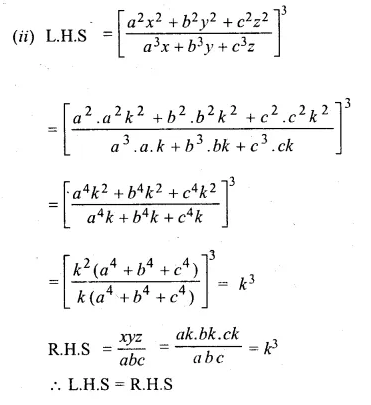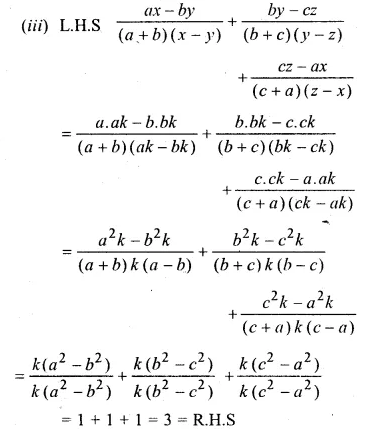Question 17.
If $$\frac { a }{ b } =\frac { c }{ d } =\frac { e }{ f }$$ prove that :
(i) (b² + d² + f²) (a² + c² + e²) = (ab + cd + ef)²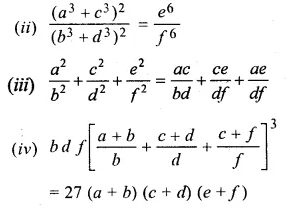Solution:
$$\frac { a }{ b } =\frac { c }{ d } =\frac { e }{ f }$$ = k(say)
∴ a = bk, c = dk, e =fk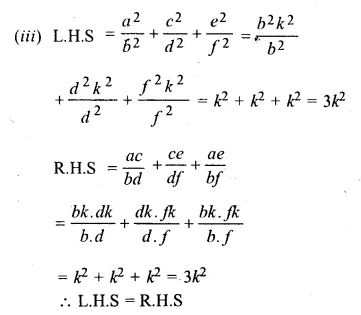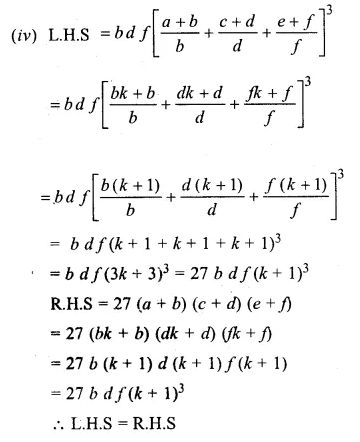Question 18.
If ax = by = cz; prove that
$$\frac { { x }^{ 2 } }{ yz } +\frac { { y }^{ 2 } }{ zx } +\frac { { z }^{ 2 } }{ xy }$$ = $$\frac { bc }{ { a }^{ 2 } } +\frac { ca }{ { b }^{ 2 } } +\frac { ab }{ { c }^{ 2 } }$$
Solution:
Let ax = by = cz = k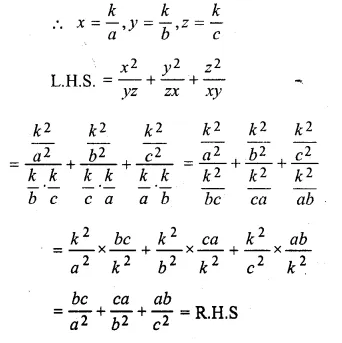Question 19.
If a, b, c and d are in proportion, prove that:
(i) (5a + 7b) (2c – 3d) = (5c + 7d) (2a – 3b)
(ii) (ma + nb) : b = (mc + nd) : d
(iii) (a4 + c4) : (b4 + d4) = a2 c2 : b2 d2.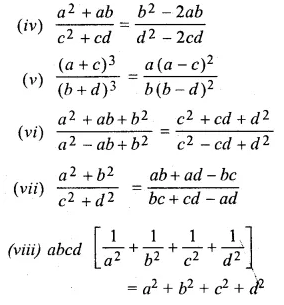Solution:
∵ a, b, c, d are in proportion
$$\\ \frac { a }{ b }$$ = $$\\ \frac { c }{ d }$$ = k(say)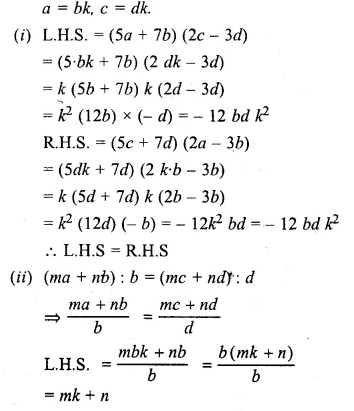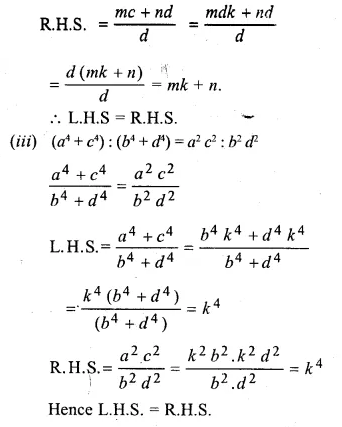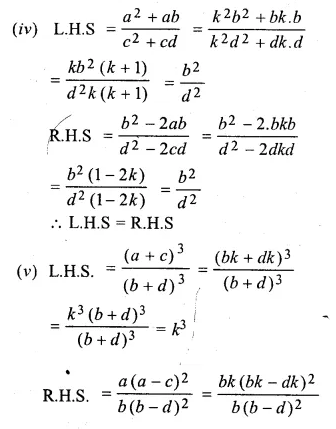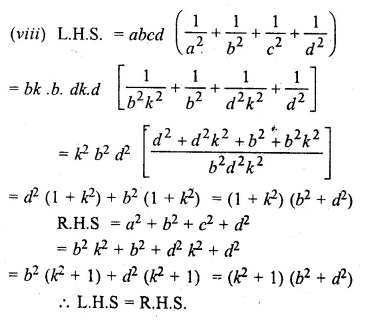Question 20.
If x, y, z are in continued proportion, prove that:$$\frac { { \left( x+y \right) }^{ 2 } }{ { \left( y+z \right) }^{ 2 } } =\frac { x }{ z }$$. (2010)
Solution:
x, y, z are in continued proportion
Let $$\\ \frac { x }{ y }$$ = $$\\ \frac { y }{ z }$$ = kQuestion 21.
If a, b, c are in continued proportion, prove that:
$$\frac { { pa }^{ 2 }+qab+{ rb }^{ 2 } }{ { pb }^{ 2 }+qbc+{ rc }^{ 2 } } =\frac { a }{ c }$$
Solution:
Given a, b, c are in continued proportion
$$\frac { { pa }^{ 2 }+qab+{ rb }^{ 2 } }{ { pb }^{ 2 }+qbc+{ rc }^{ 2 } } =\frac { a }{ c }$$
Let $$\\ \frac { a }{ b }$$ = $$\\ \frac { b }{ c }$$ = k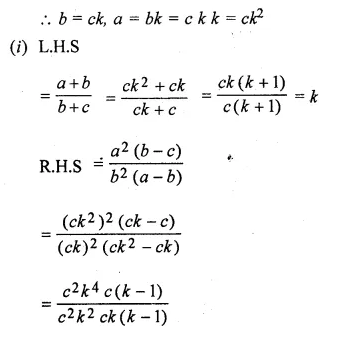Question 22.
If a, b, c are in continued proportion, prove that:
(i) $$\frac { a+b }{ b+c } =\frac { { a }^{ 2 }(b-c) }{ { b }^{ 2 }(a-b) }$$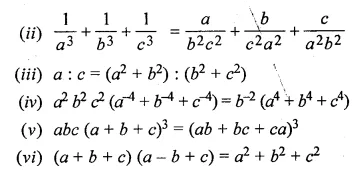Solution:
As a, b, c, are in continued proportion
Let $$\\ \frac { a }{ b }$$ = $$\\ \frac { b }{ c }$$ = k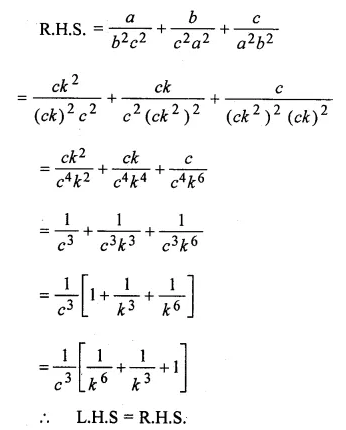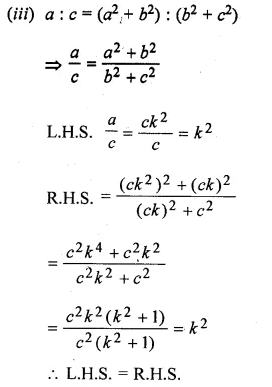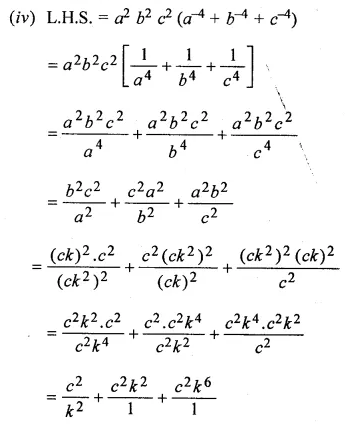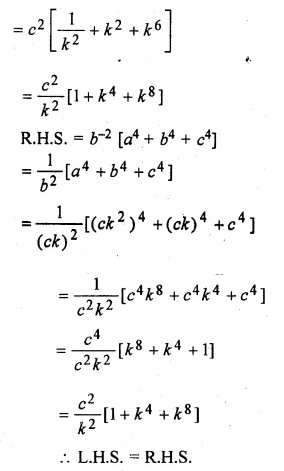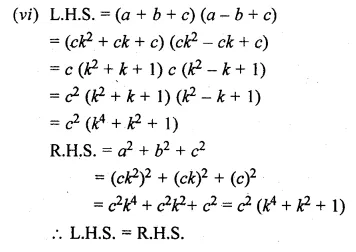Question 23.
If a, b, c, d are in continued proportion, prove that:
(i) $$\frac { { a }^{ 3 }+{ b }^{ 3 }+{ c }^{ 3 } }{ { b }^{ 3 }+{ c }^{ 3 }+{ d }^{ 3 } } =\frac { a }{ d }$$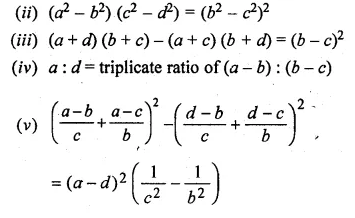Solution:
a, b, c, d are in continued proportion
∴ $$\frac { a }{ b } =\frac { b }{ c } =\frac { c }{ d } =k(say)$$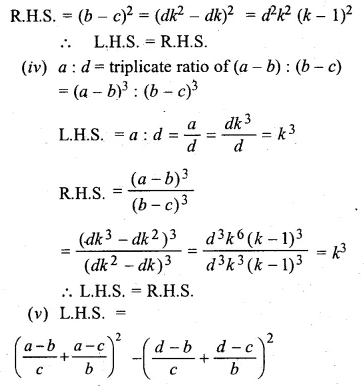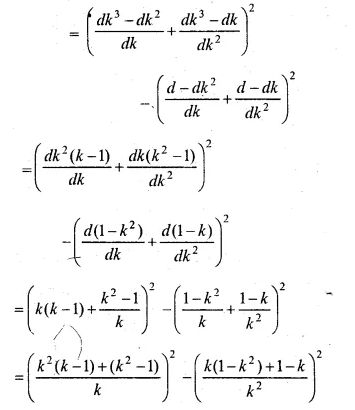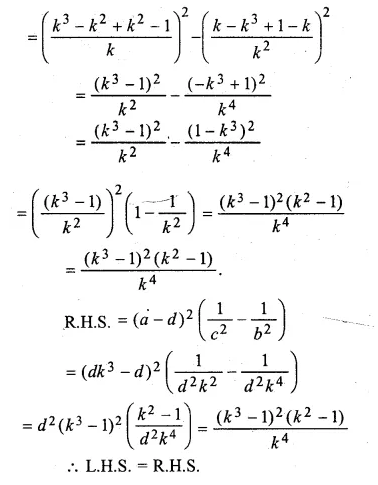Hope given ML Aggarwal Class 10 Solutions for ICSE Maths Chapter 7 Ratio and Proportion Ex 7.2 are helpful to complete your math homework.

If you have any doubts, please comment below. Learn Insta try to provide online math tutoring for you.